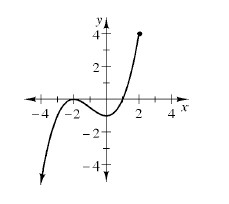### Home > AC > Chapter 12 > Lesson 12.4.2 > Problem12-79

12-79.Examine the graph of the relation at right.

1. Use inequalities to name its domain and range.

What are all the possible values of $x$, or the domain of the function?

What are all the possible values of $y$, or the range of the function?

D: $x ≤ 2$
R: $y ≤ 4$

2. Is this relation a function? How can you tell?

Does each value of $x$ have only one $y$-value?